×

×

UMD / Chemistry / CHEM 131 / When jj thomson discovered the electron, what physical property of the

# When jj thomson discovered the electron, what physical property of the Description

Exam One Study Guide

## When jj thomson discovered the electron what physical property of the electron did he measure?Chapter One

1880’s — J.J. Thompson discovers electron (e-)

1909—Robert Millikan discovers charge of electron by Oil Drop Experiment *he was able to run electric current through a metal plate to suspend oil droplets in air. He found charge to be in integer of -1.6e10^-19 coulomb.

1920—Ernest Rutherford developed Nuclear Theory through the Gold Foil Experiment. Realized that all mass of an atom and all of its positive charge is confined to the nucleus. Majority of an atom is empty space.

1932—Chadwick discovers the neutron. It reduces repulsive force between positively charged protons and therefore allows larger atoms to be stable.

SI Units

Mass, kilogram, kg

## What did rutherford discover with the gold foil experiment?Length, meter, m

Time, second, s Don't forget about the age old question of What are some of the models used to explain psychological disorders?

Temperature, kelvin, k

Electric Current, ampere, A

Amount of Substance, mole, mol

Decimal Prefixes

Deka, ten, 10

Deci, tenth, .1

Centi, hundredth, .01

Milli, thousandth .001

Micro, millionth, .0001

Formula for Average Atomic Mass = (Abundance 1 * Atomic mass 1)+ (Abundance 2 * Atomic mass 2)…+…

Chapter Two

Precision— How reproducible and close a series of measurements are to one another Accuracy— How close a measurement is to an accepted value

Random Error— Equal probability of being too high or too low

## What did james chadwick do to discover the neutron?Systematic Error— Either too high or too low

Mole→ Avogadro’s Number​. SI unit of substance. A mol contains approximately 6.02214*10^23 pieces.

Significant Figures→ In multiplication/division, use the smallest number of significant figures in the original problem for final answer rounding. In addition/subtraction, use fewest number of decimal places given in original problem for final answer rounding.

● Final answers MUST include 1) a sign 2) an amount 3) a unit 4) a label ● Treat ALL units as separate entities and perform operations on them as any other number If you want to learn more check out What are the two types of balance, and explain what they are?

Chapter Three

1) V=c/λ

2) E=hv *h is Plank’s constant = 6.626e-34(J*sec)

3) (1/λ )=R[(1/Nfinal^2)-(1/Ninital^2)] *R=1.0974e-7m Gives energy of emitted photon of light when an electron switched orbitals.

4) E of emitted photon = -hcR[(1/Nfinal^2)-(1/Ninital^2)]

5) mu^2=hu/λ *Gives wavelength of an electron-sized particle

Rh

6) En = * Gives initial energy of an orbital (shell) n2

Important Principles!

● Heisenberg Uncertainty→ The experimenter canNOT know both velocity and position of an electron. One can NOT predict the trajectory of an electron.

● Principal Quantum Number → determines size and energy of the orbital (n=1,2,3,...etc) ● Angular Quantum Number → determines shape (any value of n-1) ex. n=2, l=1,0 ● Magnetic Quantum Number→ orientation of orbital

● Spin → Electrons can only spin up or down and only with the value .5 −+ ENERGY IS PROPORTIONAL TO FREQUENCY

Properties of Light

1) Both light and electrons have wave-like and particle-like properties

2) The Bohr model shows noncontinuous energy levels “orbitals” Don't forget about the age old question of What is the difference between depth and width?

3) Heisenberg Uncertainty principle states that one can know only the position of an electron but not the speed or only the speed but not the position **Because atoms are quantized, they simply appear on one energy level and then jump to another. There is no inbetween state where an experimenter can track their movement. Don't forget about the age old question of What are the different types of effects?

4) Electromagnetic Radiation moves through space like kinetic energy. It uses waves. A magnetic vector crosses an electric vector to transfer energy in a direction. **Waves transfer energy NOT mass

Chapter Four

Relevant Periodic Groups We also discuss several other topics like What is the meaning of translation in molecular biology?

1) Group 1A = Alkali Metals *they have a charge of 1+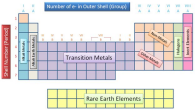2) Group 2A = Alkaline Earth Metals *they have a

charge of 2+ Don't forget about the age old question of What is the most common sensory hallucinations that occur in schizophrenia?

3) Group 7A = Halogens * they have a charge of 1-

4) Group 8A= Noble Gases * they are neutral. They don’t bond with anything. Elements are formed by nuclear reactions in stars at high temperatures.

Rules for Orbital Filling

1) Pauli Exclusion Principle → No two electrons have same four quantum number. 2) Hund’s Rule → Electrons will pair only if there is no orbital of exactly the same energy available

3) Aufbau Principle → Electrons fill in this order ; 1s,2s,2p,3s,3p….etc

EXCEPTIONS

1s,2s,3s,3p,4s,3d,4p,5s,4d,5p,6s...etc

Half-Shell Stability Effect → A closed d-orbital is more stable than a closed 4s orbital. Electrons will fill the d orbital before the s orbital in certain atoms. Ex) Cr and Cu

Notations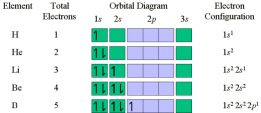1) S,p,d,f notation

2) “Orbital” box notation

3) Condensed s,p,d,f notation

→ you take the most recent

noble gas and start the notation with that in brackets. Ex) Na = [Ne] 3 s1

Valence Electrons→ Electrons involved in bonding. They are the outermost electrons. Penetration and Shielding

Coulomb's Law → E = * 1

q q1 2

4πE0r

Shielding → Creates effective nuclear charge (Z ) * Inner electrons shield outer from full eff nuclear charge

Penetration→ If outer electron gets too close to the nucleus then it will break through the protective shield of 1s orbital and it will experience full charge of nucleus. Elemental Properties

Noble Gas→ They are inert. They have full outer quantum levels and they will not bond. Metals→ They are on the left and middle of periodic table. They are malleable, ductile, good conductors, and they will lose electrons to form cations.

Nonmetals→ They are on the upper right-hand side of the periodic table. They gain electrons to form anions. They are brittle, poor conductors, and many form lighter diatomic gases. Important Periodic Trends

1) Most elements do not have an activation energy (EA) trend

2) 1A metals grow more positive down the column

3) EA is more negative as you move to the right

4) Metallic character decreases as you move to the right

5) Metallic character increases as you move down

Paramagnetic→ One or more unpaired electrons

Diamagnetic→ No unpaired electrons

Periodic Trends

1) Van der Waals Radius, for noble gases, (.5 distance between nuclei, solid phase) 2) Metallic Radius ( from metallic solids

3) Covalent Radius, for covalent molecules, (.5 distance between nuclei of bonded atoms) 4) Atomic Radius, average radius of atom computed for large number of compounds (uncertainty of 5pm)

5) **Atomic Radius ALWAYS <<<<<< van der Waals

6) Bond length = Radius of the atom (A) + Radius of the atom (B)

7) Size INCREASES down a group

8) Atomic radius INCREASES down a group

9) Atomic radius DECREASES left to right

10) Zeff INCREASES left to right

Ionization Energy→ energy required to remove an electron from element in the gas phase Binding Energy (BE) → energy required to move an electron from a specified orbital to infinite distance. It is measured using the photoelectric effect.

*** BE = hn-K.E.

Electron Affinity → A few elements GAIN electrons to form anions.

** A(g) + e- → A-(g) E.A. = ΔE

Chapter Five

Compounds→ When two or more elements combine, the new substance has different properties than the elements that formed the compound.

Covalent Bond→ Formed between two nonmetals. Electrons are SHARED instead of transferred.

Ionic Bond→ Bond between metal and nonmetal. Solid state is a lattice of alternating cations and anions.

Chemical Formula→ Indicates the number of atoms in each molecule Ex) CO2 Empirical Formula → Gives relative number of atoms in a molecule

Structural Formula→ Uses lines to show covalent bonds

Lewis Dot Structures→ The dots represent the valence electron’s. Shows depiction of the octet rule.

Nomenclature→ Naming system of compounds and molecules. The sum of (+) charges and (-) 3+ O2− Al2O3

charges must ALWAYS balance. Ex) Al and =

Examples

1) Ionic compound. Name cation metal + base name of anion +ide

a) KCL = Potassium chloride

b) CaO = Calcium oxide

2) Naming Ionic compound with a metal that has more than one type of Cation a) Fe = Iron (II) 2+

b) CrBr = Chromium (III) bromide 3

3) Naming polyatomic Ions. The charge refers to the charge of the whole ion, not each individual part.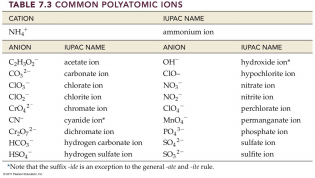a) Fe SO = Iron (II) sulfate 4

b) NH4NO3 = Ammonium

Nitrate

4) Oxy Anions are anions that contain oxygen and another element. When oxygen is more than the other element then ending = ate, when it is less, then = ite, if there are more that 2 ions = hypo is less than, per = more than.

a) ClO- = hypochlorite

b) ClO2= chlorite

c) ClO3= chlorate

d) ClO4 = perchlorate

5) Naming hydrated ionic compounds (hydrates)

a) MgSO4 * 7H20= magnesium sulfate heptahydrate

Molar Mass → [#atoms of first element][amu of first element]+[#atoms second element][amu second element]+...etc.

To find # molecules in mass given→ gCO2→ mol CO2→ # molecules

Composition of Compounds → mass % composition = element’s % of compound’s mass Equation = mass element X in one mole of compound/mass of 1 mol of compound (*100%) ● To calculate mass of element from given mass of compound. Mass of element over mass of molecule.

● *Chemical formula gives relationship between mols not mass. Mass of element in given mass of a compound → mass compound to moles compound to moles element to mass element.

To determine chemical formula from data.

1) Find mol of each element from given molecule. Divide by smallest subscript. Round to closest integer.

2) Molecular Formula = empirical formula *n (1,2,3…)

a) N = molar mass/ empirical formula molar mass

Organic Compounds

1) Organic compounds contain carbon and hydrogen. Hydrocarbons are simplest.

Exam Two Study Guide

Chapter Five

Compounds→ When two or more elements combine, the new substance has different properties than the elements that formed the compound.

Covalent Bond→ Formed between two nonmetals. Electrons are SHARED instead of transferred.

Ionic Bond→ Bond between metal and nonmetal. Solid state is a lattice of alternating cations and anions.

Chemical Formula→ Indicates the number of atoms in each molecule Ex) CO2 Empirical Formula → Gives relative number of atoms in a molecule

Structural Formula→ Uses lines to show covalent bonds

Lewis Dot Structures→ The dots represent the valence electron’s. Shows depiction of the octet rule.

Nomenclature→ Naming system of compounds and molecules. The sum of (+) charges and (-) 3+ O2− Al2O3

charges must ALWAYS balance. Ex) Al and =

Examples

1) Ionic compound. Name cation metal + base name of anion +ide

a) KCL = Potassium chloride

b) CaO = Calcium oxide

2) Naming Ionic compound with a metal that has more than one type of Cation a) Fe = Iron (II) 2+

b) CrBr = Chromium (III) bromide 3

3) Naming polyatomic Ions. The charge refers to the charge of the whole ion, not each individual part.a) Fe SO = Iron (II) sulfate 4

b) NH4NO3 = Ammonium

Nitrate

4) Oxy Anions are anions that contain oxygen and another element. When oxygen is more than the other element then ending = ate, when it is less, then = ite, if there are more that 2 ions = hypo is less than, per = more than.

a) ClO- = hypochlorite

b) ClO2= chlorite

c) ClO3= chlorate

d) ClO4 = perchlorate

5) Naming hydrated ionic compounds (hydrates)

a) MgSO4 * 7H20= magnesium sulfate heptahydrate

Molar Mass → [#atoms of first element][amu of first element]+[#atoms second element][amu second element]+...etc.

To find # molecules in mass given→ gCO2→ mol CO2→ # molecules

Composition of Compounds → mass % composition = element’s % of compound’s mass Equation = mass element X in one mole of compound/mass of 1 mol of compound (*100%)

● To calculate mass of element from given mass of compound. Mass of element over mass of molecule.

● *Chemical formula gives relationship between mols not mass. Mass of element in given mass of a compound → mass compound to moles compound to moles element to mass element.

To determine chemical formula from data.

1) Find mol of each element from given molecule. Divide by smallest subscript. Round to closest integer.

2) Molecular Formula = empirical formula *n (1,2,3…)

a) N = molar mass/ empirical formula molar mass

Organic Compounds

1) Organic compounds contain carbon and hydrogen. Hydrocarbons are simplest.

Chapter Six

6.1)

1. Morphine comes from opium poppy

2. Endorphins act as body’s painkillers

3. Valence shell electron pair repulsion (VSEPR)

6.2)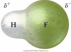1. Lewis dots show electrons as equally shared when that is not always the case. Ex) HF

a. The bond here is polar (Intermediate between

pure covalent and ionic) It is polar covalent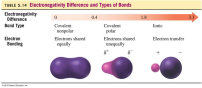2. Electronegativity increases up and to the right.

3. Greater electronegativity difference, more polar bond.

4. Dipole moment

5. Percent ionic character

a. Measured dipole moment of bond/dipole moment if electron were completely transfered (times 100%)

6.3)

1. Hydrogen atoms are always terminal

2. More electronegative elements are terminal

3. If atoms lack det/octet, form double bond.

6.4)

1. Resonance → 2 or more valid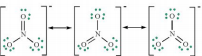structures can work. Calculate formal

charge and discriminate between

structure

2. Neutral structure is resonance hybrid

3. Delocalization lowers energy and stabilizes

4. Resonance stabilization

5. Formal charge: charge it would have if all electrons were shared equally 6. Formal charge → # valence electrons - (#nonbonding electrons + .5(#bonding electrons)) a. Sum of all formal charges in neutral molecule = 0

b. Sum of all formal charges in ion = charge of ion

c. Small (or zero) formal charges on individual atoms are better than large ones d. When formal charge can’t be avoided, negative charge should reside on most electronegative atom

6.5)

1. Exceptions for octet rule

a. Odd electron species

b. Incomplete octet

c. Expanded octet

2. Free radicals (odd # electrons)

6.6)

1. Bond energies and lengths

2. Bond energy (E) is energy required to break 1 mol of bond.

3. Single bond < double < triple bond (most energy)

4. Bond length is the average length of bond between two atoms

5. Triple is shorter than double and single

6.7)

1. Electron groups repel each other through coulombic forces

2. Repulsions from interior atoms determined geometry.

Chapter Seven

7.1)

● Paramagnetic = unpaired electrons

● Liquid oxygen is magnetic (diatomic)

● Lewis structure fails to predict magnetic character

7.2)

● Valence bond theory→ chemical bond is overlap between two half-filled atomic orbitals (AO’s)

● Hybridized atomic orbitals→ s,p,d,f orbitals

● Interaction energy is calculated as a function of internuclear distance. ● The interaction energy is usually negative (stabilizing) when the interacting atomic orbitals contain a total of two electrons that can spin-pair (orient with opposing spins) ● The geometry of overlapping orbitals determines shape

7.3)

● Orbitals in a molecule aren’t the same as orbitals in an atom.

● Hybridization → standard atomic orbitals combined to form hybrid orbitals. ● Greater the overlap, the lower the energy and the stronger the bond is. ● Hybrid orbitals minimize energy by maximizing orbital overlap.

● The number of standard atomic orbitals = the number of hybrid orbitals

● sp orbital 3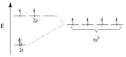● **Nitrogen orbitals in ammonia are sp hybrids. 3 ● sp → has one s and two p orbitals and one unhybridized p orbital. Trigonal planar. 2 ● When p orbitals overlap side to side it makes a π bond. When they overlap end to end they make a sigma σ bond.

● Single = sigma

● Double = one sigma and one pi

● Triple = two pi and one sigma

● Compounds with same molecular formula but different structures are ISOMERS.

7.4)

● Linear combination of atomic orbitals = a weighted linear sum- analogous to a weighted average- of the valence atomic orbitals of the atoms in the molecule.

● Bonding orbital = lower energy and increased electron density

● Antibonding= raises energy and decreases electron density.

● Different phases result in destructive interference.

● Bond Order = [# electrons in bonding molecular orbital - # in antibonding molecular orbital]/2

● Order =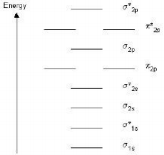7.5)

● Electrons delocalize over the entire molecule

7.6)

● Electron sea→ When metal atoms donate greater than or equal to one electron. ○ Conductivity

○ Malleability

○ Ductile

● Semiconductors and Band Theory

○ Spacing gets small and it is just a band of energy levels

○ Bond Gap is large in insulators

○ Doping→ “holes” in valence bonds

○ Silicon and Phosphorus → n-type semiconductor

○ P-type → holes move opposite to electrons

○ P-n → junctions = diodes and amplifiers

Chapter Eight

8.1)

● Carbon dioxide amounts are growing

● Greenhouse gas effects → cause global warming

8.2)

● Chemical change → atoms rearrange and transform original substances into different. Ex. burning gasoline. Properties → corrosiveness, flammability, ductility, toxicity. ● Physical → Do not change composition. Ex. boiling water. Properties → Odor, taste, appearance, melting, boiling point, density.

● Chemical changes are evidenced by temperature and color change.

8.3)

● Chemical reactions are made of reactants on the left and products on the right.

● The equations must be balanced

● Hint** Balance the most complex substance FIRST.

8.4)

● Coefficients in a chemical equation specify the relative amounts of moles of each of the substances involved in the reaction.

● Stoichiometry→ Relationship between chemical amounts. USE RATIOS ● Mass→ moles→ moles (ratio) → mass

8.5)

● Limiting reactant, Theoretical yield, Percent Yield.

● Limiting reactant → (reagent) completely consumed and limits the amount of product ● Reactant in excess → greater quantity than is required

● Theoretical Yield → product that can be made based on limiting reactant . ● Actual Yield → Actual amount of produced yielded. (actual/theoretical)*100% 8.6)

● Combustion reaction involves a substance and oxygen (diatomic molecule) and it emits heat, water, and carbon dioxide.

● Alkali → Reacts with halogens. More heat and stronger down group 1A ● Halogens → Make (metal halides), ionic bonds, also reacts with hydrogen to make hydrogen halides… Forms covalent bonds

● Forms interhalogen bonds.

Chapter Nine

9.1)

● Molecular gastronomy → chemical cooking

● Precipitation reaction → solutions, homogeneous mixtures often containing a solid dissolved in a liquid are mixed.

9.2)

● Solution Concentration

○ Homogeneous mixture = solution. Major component is solvent, minor = solute. Aqueous = water is the major component.

○ Dilute versus concentrated solution.

○ Molarity (M) = amount solute(mol)/volume solution (L)

○ Stock solution (concentrated form)

○ M1V2=M2V2

9.3)

● Solution Stoichiometry

○ Volume A → Amount A→ Amount B→ Volume B

9.4)

● Types of Aqueous solutions and solubility

○ Solution salt= electrolyte

○ Solution sugar = nonelectrolyte

○ Dissolve and conduct = electrolyte

○ Sodium dissolves into ions = strong electrolyte

○ Molecules don’t dissociate = non electrolyte

○ Strong acid = strong electrolyte

○ Weak acid = weak electrolyte

9.5)

● Only insoluble compounds form precipitates

9.6)

● Aqueous Reactions: MOlecular, ionic, and complete ionic.

● You can write complete ionic equations

● Spectator ions→ don’t participate in reaction

● Net ionic reactions -> show only changes.

● MOlecular → Chemical equation showing complete, neutral formulas for every compound in a reaction.

● Complete ionic equations → show all species as if they are actually present in solution ● Net ionic equation ---> shows only species that change in reaction

9.7)

● Acid Base Reactions

○ Acid-base = neutralization reaction

○ Acid reacts with base and produces water

○ Arrhenius definition

○ Polyprotic acids → contain more than one ionizable proton and release them sequentially.

○ Bases produce OH- in solutions

○ Acids → Binary/Oxyacids.

○ Oxyacids → ate-ic and ite-ous

○ Acid base form water and ionic compound (salt)

○ Acid base titration → equivalence point

○ # moles of OH- = #moles of H+ in solution

○ Indication (dye) depends on acidity

9.8)

● Gas evolution reaction s→ gas bubbles

○ Anion combines with cation

○ Intermediate products

9.9)

● Oxidation - Reduction Reactions

○ Redox reactions → electrons transfer from 1 reactant to the other. Manny involve O2.

○ Oxidation = loss of electron

○ Reductions gain electrons

○ OXidation States

■ Oxidation # to atom based on electron assignments

■ Oxidation # of atom in compound is the “charge” it would have if

all the shared electrons were assigned to the more electronegative

atom

● Oxidation state free atom == 0

● Oxidation state of monoatomic = charge

● Sum of oxidation states of all atoms in a neutral molecule

or formula unit = 0

● In an ion = charge of ion

● Metals have positive oxidation states

■ In redox reaction

● Oxidizing agent oxidizes another substance (and it is

reduced)

● The reducing agent reduces other substances (itself is

oxidized)

In Class

1. Acids → That which produces H+ (more precisely H30+) ion in water

a. Two types. Binary acids (HCL, HBr) Oxy acid (HNO3, H2SO4, HClO4) i. Nearly all the rest are weak acids

2. Base→ That which produces OH- in water

a. Most are water soluble hydroxides

b. Reactive anions (high charge density)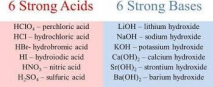1. For binary diatomic acids, acid strength = f(ΔEN) & bond length

a. Strong acids ionize completely

b. For except for HF, Δ EN are .6 to .9, so HS’s are moderately polar, not “ionic”

c. Longer bond = stronger acid d. HF < HCl < HBr < HI

Chapter 10

10.1)

● Combustion of ethanol is an exothermic reaction

● Water evaporation is endothermic

● Thermochemistry is the relationship between chemistry and energy

● Chemical reactions exchange energy with surroundings

10.2)

● Energy is the capacity to do work

● Work is the result of a force acting through a distance

● Energy can be transferred through heat

● Kinetic energy is the energy associated with motion

● Thermal energy is the energy associated with temperature

● Potential energy is the energy associated with position or composition ● Chemical energy is the energy associated with the relative positions of electrons and nuclei in atoms

● Joule (J) is (kg*m^2)/s^2

● Calorie = 4.184 J

● Calorie = 1000 calories = 1kcal

● Law of conservation of energy states that energy can’t be created nor destroyed ● To track energy, look at the system and its surroundings

10.3)

● Thermodynamics is the study of energy and its conversions

● The first law states that the total energy of the universe is constant

● Internal energy is the sum of kinetic and potential energy of all the particles that compose the system.

● Internal energy is a state function, it depends on the state of the function, not on how the system arrived at the state

● ΔE = Efinal − Einitial ΔE = Eproducts − Ereactants

● If ΔE is negative then energy flows out of the system into the surroundings ● If ΔE is positive, then energy flows into the system out of the surroundings ● ΔE = q+w q= heat w= work

● If q is positive then the system gaints thermal energy, if q is negative then the system loses thermal energy

● Positive w means work is done on the system, negative w means work done by the system

10.4)

● Temperature is the measure of thermal energy, heat is the transfer of thermal energy ● Thermal energy flows from hot to cold

● Thermal equilibrium means no net transfer of heat

● ΔT = Tfinal-Tinitial

● Q is proportional to heat

● q= C(heat capacity) times ΔT

● Heat capacity is J/degrees C and it is an extensive property

● Measure of intrinsic capacity to absorb heat is (Cs) the specific heat capacity which is J/gC and molar is J/molC

● Heat → q = m x Cs x ΔT

● Pressure-volume work is the force that is caused by volume change against external pressure

● w= -PΔ V the units are L*atm 101.3 J = 1L*atm

10.5)

● Calorimetry measures thermal energy of a reaction and its surroundings. Qrxn = -Qcal 10.6)

● Enthalpy is the sum of internal energy and product of its pressure and volume. ΔH = ΔE + PΔV

● The value of ΔH is the amount of heat absorbed or evolved in the reaction under constant pressure

● Potential energy is the source in an exothermic reaction

● Bonds break and release energy and energy is absorbed when bonds form 10.7)

● Bomb calorimetry occurs at constant volume and measures ΔE for a reaction ● Coffee-cup calorimetry occurs at a constant pressure and measures ΔH for a reaction 10.8)

● Relationships between chemical equations and ΔH rxn

● If a chemical equation is multiplied by some factor then so is ΔH rxn ● If an equation is reversed then the sign of ΔH rxn is switched

● ΔH for the overall equation is the sum of the reaction heat for each step. This is called Hess's Law

10.9)

● We can estimate ΔH from bond energies

● ΔH rxn = ΣΔHbond′s broken + ΣΔHbond′s formed

● A reaction is exothermic when weak bonds break and strong bonds form ● A reaction is endothermic when strong bonds break and weak bonds form ● Bond formation always releases energy

10.10)

● Standard for enthalpy

○ Standard state, standard enthalpy change, standard enthalpy of formation ● Standard state

○ Gas → pure gas at 1atm

○ liquid/solid → most stable form at 1 atm and temperature of interest ○ Solution → concentration of 1 M

● Standard enthalpy of chang

○ Process when all the reactants and products are in standard states

● Standard enthalpy of formation

○ Pure compound→ change when 1 mol forms its constituent elements in their standard states

○ Pure element → ΔH f = 0 in standard state

● Elements → compounds is positive ΔH f

● Compounds → elements is negative ΔH f

● To calculate ΔH rxn, subtract the enthalpies of formation of the reactants multiplied by their stoichiometric coefficients from the enthalpies of formation of the products multiplied by their stoichiometric coefficients.

Chapter Eleven

11.1)

● Pressure is a force exerted per unit by area by gas particles as they strike each other and surfaces around them

11.2)

● Pressure = force/area = F/A

● Variation in pressure creates wind

● Pressure decreases with increasing altitude

● Millimeter of mercury → mmHg or torr

● Atmosphere = atm. 1atm is sea level and standard pressure. 1 atm= 760 mmHg ● SI unit of pressure is pascal (Pa). Newton per meter squared. 101325 Pa = 1 atm ● Manometer is a U-shaped tube containing dense liquid.

11.3)

● Basic gas properties. Moles, volume, pressure, and temperature

● Boyle’s law. P1V1=P2V2

● Charles’ law. (V1/T1=V2/T2)

● 0K = -237.15 degrees Celsius

11.4)

● Ideal gas law. PV=nRT

● R=.08206 L*atm/mol*K

11.5)

● Volume occupied by one mole of a substance is its molar volume. Standard temperature and pressure are 273K and 1 atm. The volume is 22.4L

● Density of a gas is molar mass/ molar volume

● You rearrange PV=nRT to find moles and then find molar mass

11.6)

● Partial pressure is the pressure die to any individual component in a gas mixture (Pn) ● Ptotal = Pa+ Pb+ Pc….etc

● Dalton’s law of partial pressure

● Mole fraction is Xa. Xa= Na/Ntotal

● Pa=XaPtotal

● Hypoxia is oxygen starvation

● Oxygen toxicity if atm is greater than 1.4

● Nitrogen narcosis makes you feel drunk

● Scuba divers have a special mixture of air

● Partial pressure of water in a mixture is vapor pressure.

● Vapor pressure increases with increasing temperature.

11.7)

● Kinetic molecular theory. Gas is modeled as a collection of particles in constant motion ○ The size of a particle is negligible small

○ The average kinetic energy of a particle is proportional to the temperature in kelvins.

■ Kinetic energy = .5mv^2

■ The helium atoms are lighter and they must move faster to have the same kinetic energy as the heavier atoms.

■ The collision of one particle with another is completely elastic particles exchange energy, but there is no overall loss of energy

11.8)

● In a gas mixture at a given temperature, the lighter particles travel faster than heavier ones. = Urms √ M

3RT

11.10)

● Stoichiometry with gases

● Mass a→ moles a→ moles b→ mass b

● Find moles by rearranging ideal gas law

● 1 mol ideal gas= 22.4 L, this can be used as a conversion factor

Chapter 12

12.1)

● Isomers, same chemical formulas but different compounds. Structure determines properties

● Intermolecular forces → attractive forces that exist between all molecules and atoms ● If thermal energy is high compared to intermolecular, it's a gas and visa versa 12.2)

● Solids and liquids are more dense than gas

● Molecules in liquids and solids are close while gas molecules are farther apart ● Solid water is less dense than liquid

● Liquids are not easily compressed

● Solids can be crystalline or amorphous

● Delta temperature or delta pressure can change states of matter

● Sorting gases liquids is efficient because it takes less space

12.3)

● Intermolecular forces are weaker than bonding.

● Dispersion force → the results of fluctuations in electron distribution in atoms or molecules. Instantaneous dipole/temporary dipole

● Magnitude of dispersion force depends on how easily electrons polarize ● Boiling point of n-alkanes increases with molar mass

● Dipole-dipole force exists in polar molecules.

● Permanent dipole → electrons are dense along one element in molecule ● Polar have higher melting and boiling points than nonpolar

● Miscibility → the ability to mix without separating two states “like dissolves like” ● Hydrogen bonding

● H-bond is super dipole-dipole force

● Hydrogen bonds differ from chemical bonds which occur between individual atoms within a molecule. H-bonds occur between molecules.

● H-bonds are weaker than covalent bonds

● Ion-dipole force → occurs when ionic compound is mixed with a polar compound. This is the strongest intermolecular force

12.4)

● Surface tension → tendency of liquid to minimize their surface area. Energy required to increase surface area by unit amount.

● Surface tension decreases as intermolecular forces decrease

● Water drop is sphere in antigravity → smallest surface area to volume ratio ● Viscosity → resistance of a liquid to flow.

○ Measured in poise (P)

● Increasing viscosity with increasing molar mass

● Liquids become less viscous as temperature increases

● Capillary action → ability of liquid to flow against gravity up a narrow tube ● Cohesive forces (attraction between molecules) and adhesive (between sides of tube)

12.5)

● Vaporization→ the process by which thermal energy can overcome intermolecular forces and produce a state change from liquid to gas.

○ The higher the temperature, the greater the average energy of the collection of molecules.

● Condensation is the opposite of vaporization.evaporation takes place at a faster rate than condensation.

● Liquids that vaporize easily are volatile.

● Vaporization increases with increasing temperature.

● The rate of vaporization increases with increase=in surface area

● The rate of vaporization increases with decreasing strength of intermolecular forces ● Vaporization is an endothermic process.

● Amount of heat required to vaporize one mole of a liquid to a gas is its HEAT OF ENTHALPY OF VAPORIZATION. This number is always positive

● Dynamic equilibrium is when the rate of condensation and vaporization is equal. ● The pressure of a gas in dynamic equilibrium is vapor pressure

● When a system in dynamic equilibrium is disturbed, the system responds so as to minimize the disturbance and return to a state of equilibrium.

● Le Chatelier’s Principle

● Boiling point of a liquid is the temperature at which the liquid’s vapor pressure equals the external pressure.

● Normal boiling point is when the temperature at which its vapor pressure equals 1 atm. ● Clausius-Clapeyron equation

● Supercritical fluid is the substance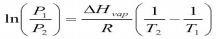which offers when the temperature is

the critical temperature. The liquid

cannot exist. The pressure is called the critical pressure.

12.6)

● Sublimations is from solid to gas and deposition is gas to solid.

● Melting point. The molecules have enough energy to overcome intermolecular forces and the solid turns to liquid. The opposite is freezing. Heat of fusion is the amount of heat required to melt 1 mole of a solid. Melting is endothermic

● Heat of fusion is less than heat of vaporization.

12.7

● Heating curve of water

12.8)

● Water has a low molar mass yet it is liquid at room temperatures. Bent structure makes it very polar. Water expands upon freezing which is unusual.

Chapter 16 Notes

16.1)

● Hemoglobin reacts with oxygen

● Double arrow means reaction can reach equilibrium

● (K) is equilibrium constant. Small (k) means reaction lies to the left and visa versa ● Hemoglobin binds oxygen when the surrounds have high O2 and releases when surrounds are lacking

16.2)

● Reactions that can occur in both directions are reversible

● Dynamic equilibrium for a chemical reaction is the condition in which the rate of the forward reaction equals the rate of the reverse reaction

● At equilibrium in the same reactions, the concentrations of the reactants and products are not equal to one another

16.3)

● Rates of forward and reverse reactions are equal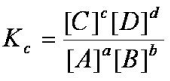● Law of mass action

● Coefficients become exponents

● k>1, shift to products, proceeds to completions

● k<1, shift to reactants, reaction does not proceed far

● k=1, at equilibrium, reaction proceeds halfway

● If you reverse the reaction then you invert k value

● If you multiply then you raise k to that same power

● If you add equations (elimination) then you multiply corresponding k values 16.4)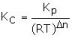● The expression for Kp takes form of Kc expect partial pressure replaces concentrations

● K does not have units, they cancel out

16.5)

● In a solid, concentration depends on density

● Pure solids and liquids are not included into the equation

16.6)

● I.c.e. Table. Initial, change, equilibrium

16.7)

● Qc is the reaction quotient

● It depends on current state of reaction, like temperature

● The value of Q relative to K is a measure of the progress of the reaction towards equilibrium.

● q<k, shift towards products

● q>k, shift towards reactants

● q=k, at equilibrium

16.8)

● Finding equilibrium, concentrations

● Sometimes you need quadratic equations

● Method o f successive approximation (x<5%)

16.9)

● When a chemical system at equilibrium is disturbed, the system shifts in a direction that minimizes the disturbance

● Le chatelier's’ principle

● Increasing reactants gives a shift to the right

● Increasing products gives a shift to the left

● Decreasing reactants shifts to the left

● Decreasing productus shifts to the right

● Decreasing volume causes reaction to shift to the direction that has fewer moles of gas ● Increasing volume causes shift to greater number moles of gas

● Adding inert gas has no effect

● If reaction has equal number of moles then changing the volume does nothing ● In an exothermic

○ >T, shift to left

○ <T, shift to right

● In an endothermic

○ <T, shift to left

○ >T, shift to right

Chapter 17

17.1)

● Water dilutes concentrated acids and prevents additional burns

17.2)

● Acids → sour, ability to dissolve metal, turns blue litmus paper red, neutralizes bases ● HCl is the main component of stomach acid

○ Acetic acid is in vinegar

○ Carboxylic acid is found in substances derived from living organisms ● Bases → bitter, slippery, turn red litmus paper blue, neutralize acids ● Alkaloids, organic bases in plants that are poisonous.

17.3)

● Arrhenius definition

○ acid→ substance that produces H+ ions in aqueous solution

○ Base → substance that produces OH- in solution

○ In water H+ ions bond and form H3O+, hydronium ion

○ Strong acid completely ionizes, weak acids partially ionize. Single arrow versus double arrow. Same with strong base

○ Acids and bases form water and neutralize each other

● Bronsted-Lowry definition, focuses on the transfer of H+ ions

○ Acid-proton donor

○ Base- proton acceptor

○ The donors and acceptors are always occurring together

○ Some substances can act as both (amphoteric)

○ Conjugate acid-base pair

○ Conjugate acid is any base to which a proton has been added

○ A base accepts a proton and is a conjugate acid

○ An acid donates a proton and becomes conjugate base

17.4)

● Binary acids

● Factors affecting ease with which hydrogen is donated are polarity and strength of bond ● Hydrogen must be positively charged so consider dipole

● Stronger bonds are weaker acids, because harder to dissociate

● Oxyacids, hydrogen bonded to an oxygen, which is bonded again

● If oxyacids contain additional oxygen atoms then it is a stronger acid 17.5)

● Autoionization of water and pH

● Water can react with water and form H3O+ and OH- kw= equilibrium constant is 1.0*10^-4, in a neutral solution

● In an acid solution, h+>OH- and visa versa

● Neutral solution, the H+ = OH-

● pH is the -log of the hydronium ion

● Sig figs are 2 decimals if original used 2

● pH <7 is acidic, = 7 is neutral, >7 basic

● pOh is the -log of hydroxide ion

● pH + pOH = 14

● pKa=-logKa, smaller pKa = stronger acid

17.7)

● In a strong acid, the concentration of hydronium is = to the concentration of the acid ● Determining pH of a weak acid is harder because concentration of weak acid doesn’t equal concentration of hydronium

● Use ICE table to find the Ka of hydronium

● Percent ionization of weak acid is the concentration of hydronium/ inition acid concentration +100%

● % is usually very small <5%

● Equilibrium concentration of hydronium in a weak acid increases with increasing initial concentration of the acid and visa versa

● Strong acid and weak acid

● The ionization of a strong acid prevents the weak acid to ionize less than it would ● If you're solving for Ka of weak acid, you can’t neglect the initial concentration of H3O+ ● A mixture of 2 weak acids can negate the one with a smaller Ka

17.8)

● Double arrow indicates ionization isn’t complete

● pKb=-logKb

● You find it the same as Ka

● Neglect weak base

● You find (M) weak base the same as acid

17.9)

● Anions tend to form either basic or neutral solutions while anions form acidic or neutral solutions

● Anion is the conjugate base of a weak acid

● Anion that is the conjugate base of a strong acid is neutral

● To find pH of solution containing an anion acting as a weak base you need Kb for anion which you figure out from ka

● kb=kw/ka

● pka+pkb=14

● Cations as weak acids

○ Cations are counterions of strong bases because they are pH neutral

○ Cations that are conjugate acid of a weak base is a weak acid

○ Small highly-charged metal cations form weak acid solution

○ Salts in which neither the cation or anion acts as an acid base from pH neutral solutions.

○ Salts in which the cation doesn’t act as an acid and the anion acts as a base are basic solution and visa versa

○ Salts which cation acts as acid and anion acts as base form solution where pH depends on relative strength of acid and base

17.10)

● Polyprotic ionizes in 2 steps.

● Ka is acidic ionization and Ka2 is acid ionization constant for the second step (it is smaller)

● pH, you only treat the first Ka as important

● To find concentration of k2 you must use concentration of Ka as initial in ice chart.

17.11)

● Lewis model. Focuses on donation of electron pair

● acid→ accepts

● Base → donates

● A lewis acid has an empty orbital that can accept an electron pair

● It expands what can be classified as an acid

● Water is lewis acid

● Some cations can act as acids because they are positively charged and have empty orbitals

Page ExpiredIt looks like your free minutes have expired! Lucky for you we have all the content you need, just sign up here